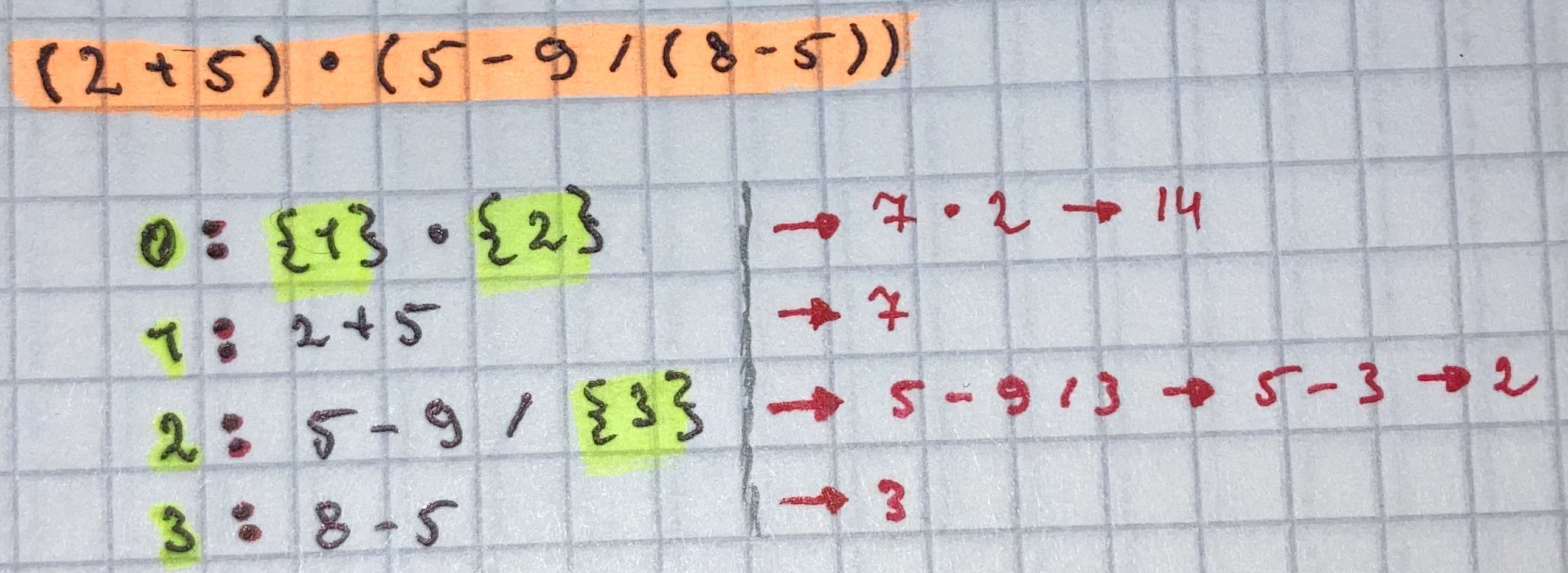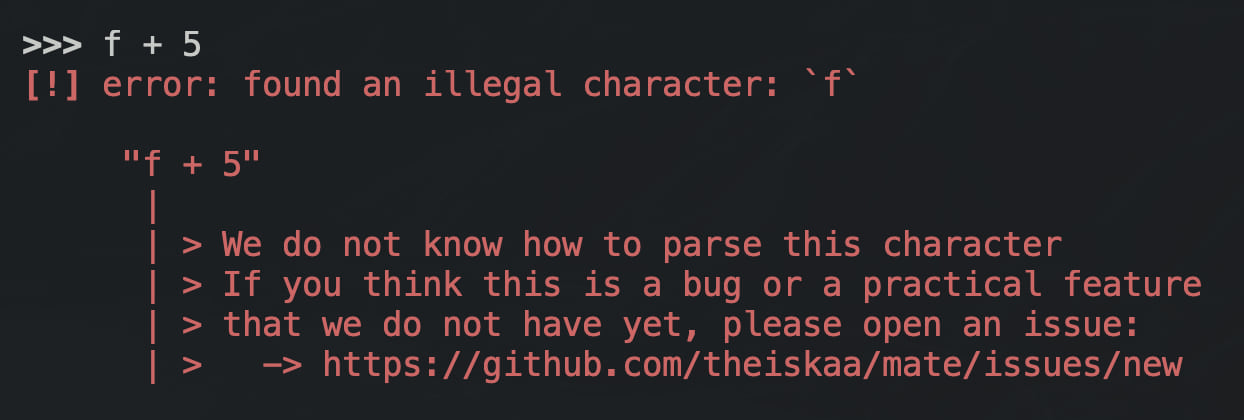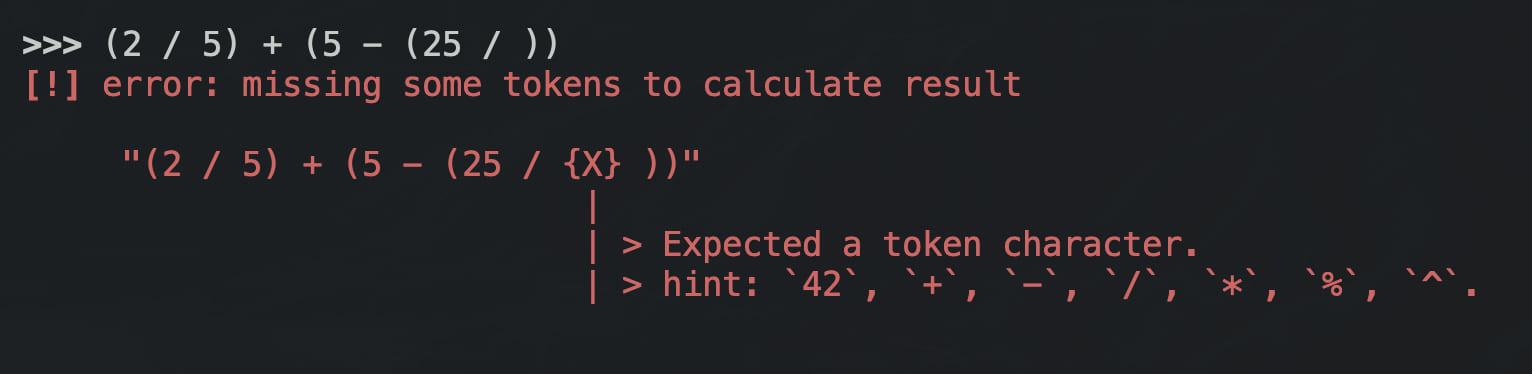## mate-rs

A simple and lightweight arithmetic expression interpreter

### 5 releases

 0.1.4 Nov 2, 2022 Sep 28, 2022 Sep 25, 2022 Sep 23, 2022 Sep 19, 2022

#73 in Math

110KB
2K SLoCMate is a library for parsing and calculating arithmetic expressions inputted as &str(string). Uses Lexer(similar to interpreted-programming languages' lexer) structure to parse string input in to token list, and Calculator structure to calculate final result from token list. Implements also a general wrapper structure that implements Lexer and Calculator inside of it. And makes it easy to calculate arithmetic expression's result directly without dealing with parsing and calculating manually.

# Usage

This crate is on crates.io and can be used by adding mate-rs to your dependencies in your project's `Cargo.toml`.

``````[dependencies]
mate-rs = "0.1.4"
``````

## Example: with `Mate`

`Mate` is general wrapper structure for `Lexer` and `Calculator`. has only one method that used to `calculate` result via string(&str) input.

``````use mate_rs::mate::Mate;

let result = Mate::calculate("6 * 7");
match result {
Ok(v) => assert_eq!(v, 42.0),
Err(_) => {
// Do something ...
}
};
``````

## Example: with `Lexer` and `Calculator`

`Lexer` is the main structure that parses string-input to token-list. `Calculator` is the structure that used to calculate final result via `Lexer`'s result.

``````use mate_rs::{calculator::Calculator, lexer::Lexer};

// Generated tokens gonna be something like:
//  |
//  | Token(
//  |   type: SUBEXP,
//  |   tokens: [
//  |        Token(
//  |          type: SUBEXP,
//  |          tokens: [
//  |               Token(type: NUMBER,  literal: "2")
//  |               Token(type: PLUS,    literal: "+")
//  |               Token(type: NUMBER,  literal: "5")
//  |          ],
//  |        ),
//  |        Token(type: PRODUCT, literal: "*"),
//  |        Token(
//  |          type: SUBEXP,
//  |          tokens: [
//  |               Token(type: NUMBER,  literal: "5")
//  |               Token(type: MINUS,   literal: "-")
//  |               Token(type: NUMBER,  literal: "9")
//  |               Token(type: PLUS,    literal: "+")
//  |               Token(
//  |                 type: SUBEXP,
//  |                 tokens: [
//  |                      Token(type: NUMBER,  literal: "8")
//  |                      Token(type: PLUS,    literal: "-")
//  |                      Token(type: NUMBER,  literal: "5")
//  |                 ],
//  |               ),
//  |          ],
//  |        ),
//  |   ],
//  | ),
//  | Token(type: PLUS,    literal: "+")
//  | Token(type: NUMBER,  literal: "35")
//  |
let input = "[ (2 + 5) * (5 - 9 + (8 - 5)) ] + 35";
let tokens = Lexer::lex(input.clone()).unwrap(); // should handle error case also

// Result will be calculated from tokens, by X/O/Y algorithm.
//
//  ╭────────╮ ╭───────────╮ ╭────────╮
//  │ NUMBER │ │ OPERATION │ │ NUMBER │
//  ╰────────╯ ╰───────────╯ ╰────────╯
//       ╰───╮       │        ╭───╯
//           ▼       ▼        ▼
//           X  [+, -, *, /]  Y
//
let result = Calculator::calculate(tokens, input.clone());
``````

# How it works

Mate is all about two main structures, [Lexer] and [Calculator]. [Lexer] is the structure that takes care of parsing interpretting given string expression, and [Calculator] is the structure that takes care of calculating final result via parsed tokens.

## Lexer

Loops through the given input string, reads and converts each character to an [Token] structure. We've several types of main tokens and they are:

• `ILLEGAL` - illegal character.
• `NUMBER` - number type.
• `MINUS`, `PLUS`, `PRODUCT`, `DIVIDE`, `PERCENTAGE`, `POWER` - operations.
• `LPAREN`, `RPAREN` - parentheses.
• `LABS`, `LPAREN` - absolute values.
• `SUBEXP` - sub expression, expressions inside of parentheses, abs, or combinations of division and multiplication.

Lexer's `lex` functionality converts each character to one of these tokens. It combines multiplication or division operation related tokens into one sub-expression to keep the operation-priority right. And nests the parentheses, absolute values and powers with a custom level-to-expression algorithm.

level-to-expression algorithm is mapping algorithm that maps concrete expression to it's nesting level.

For example if the given token list is -> `(2 + 5) : (5 - 9 / (8 - 5))`. Generated result will be:By doing that we make it easy to keep the operation-priority safe.

## Calculator

Calculator takes the parsed token-list and calculates final result of it. Uses custom `X/O/Y` algorithm a.k.a `X/OPERATION/Y` where `X` and `Y` are numbers, and `O` is operation. If cannot get the `X` or `Y` takes them as zero.

``````╭────────╮ ╭───────────╮ ╭────────╮
│ NUMBER │ │ OPERATION │ │ NUMBER │
╰────────╯ ╰───────────╯ ╰────────╯
╰───╮       │        ╭───╯
▼       ▼        ▼
X  [+, -, *, /]  Y
``````

# Syntax

The syntaxt of `Mate` kept as easy and basic as possible. It's just plain-text mathematics syntaxt with few customizations. Let's see some examples:

### Plus and Minus

`2 + 5` and `2 - 5` are valid syntaxts. (in `x <operation> y` where x and y could be `NUMBER` or `SUBEXP`).

### Multiplication, Division and Percentage

`4 * 2`, `4 / 2`, and `4 % 2` are valid syntaxts. (in `x <operation> y` where x and y could be `NUMBER` or `SUBEXP`). Also `4(10 / 2)` or `4(2)` is valid syntax in case of multiplication.

### Power

`4 ^ 2` is valid syntaxt. (in `x <operation> y` where x and y could be `NUMBER` or `SUBEXP`). Also continues powers are accepted: `4 ^ 2 ^ 3` which gonna be automatically turned into `4 ^ (2 ^ 3)`.

### Parentheses

`(2 * 5)` is valid syntaxt. (in `x <operation> y` where x and y could be `NUMBER` or `SUBEXP`). And, nested parentheses expressions are accepted: `(2 * ((5 * 2) / (9 - 5)))`.

### Absolute-Values

`[2 - 12]` is valid syntaxt. (in `x <operation> y` where x and y could be `NUMBER` or `SUBEXP`). And, nested absolute-value expressions are accepted: `[7 - 14] * [5 - 9 / [5 - 3]]`.

# Errors

#### We do not need to say anything about errors, here is some of them:~0.9–1.3MB
~37K SLoC